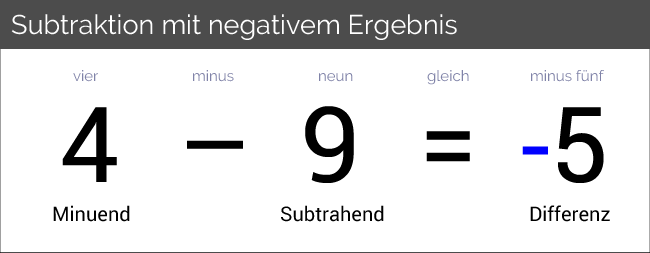# 1 9 is an integer

## Whole numbers

Subtracting two natural numbers can result in a number smaller than:The result is always a negative number if the subtrahend is greater than the minuend.

For example, the result is that this is a negative integer. This number does not belong to the natural numbers as they are all positive. Therefore we introduce a new set of numbers, which we call the whole numbers call.

The whole numbers are necessary because the natural numbers cannot represent negative values.

Negative values ​​(i.e. values ​​less than) are required in everyday life, for example, for: Altitudes, temperatures, account balances, etc.

By the way, the word “negative” here means “opposite” (the opposite of the positive numbers).

The whole numbers are the natural numbers and their inverse, including the number zero. “Inverse” means that all natural numbers are given a minus (negative sign).

The formula sign of the whole numbers is and the amount is written down as:

The enumeration of the numbers can be continued as long as you like in both directions. Every whole number always has a predecessor (with) and a successor (with). So there are infinitely many whole numbers.

If we look at the predecessors, we go in the direction of minus infinity (). When we consider the successors, we are going towards plus infinity ().

The natural numbers are considered a subset of the whole numbers, we write.

### Set notation

We use the symbol ∈ to represent belonging to a set and the symbol ∉ to express that the element does not belong to a set. Examples:

("5 is an element of the whole numbers")
("-77 is an element of the whole numbers")
("0.5 is not an element of the whole numbers")

There are other number ranges that include allow a division that leaves a remainder. Compare this with rational numbers.